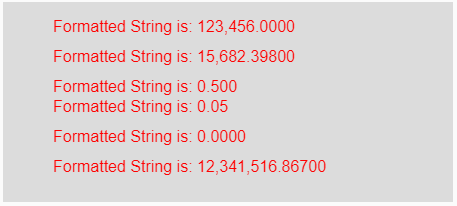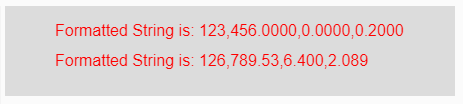Related Articles
p5.js | nfc() function
• Last Updated : 22 Apr, 2019

The nfc() function in p5.js is used to format the input numbers (integers or floats) into strings as well as it places appropriate commas (,) to mark the unit of 1000. It contains two versions, first is used to formatting the integers and second is used to formatting the array of integers.

Syntax:

`nfc( Num, Right )`

Parameters: This function accepts two parameters as mentioned above and described below:

• Num: This parameter holds the number or string or array of numbers which are to be formatted and placing the appropriate commas (,) to mark the unit of 1000.
• Right: This is the positive number which says the number of digits should be on the right side of the decimal point.

Return Value: It returns the formatted string.

Below programs illustrate the nfc() function in p5.js:

Example 1: This example uses nfc() function to format the input numbers into strings as well as it places appropriate commas to mark the unit of 1000.

 `function` `setup() {  ` `  `  `    ``// Creating Canvas size ` `    ``createCanvas(450, 200);  ` `}  ` ` `  `function` `draw() {  ` `      `  `    ``// Set the background color  ` `    ``background(220);  ` `    `  `    ``// Initializing the Numbers ` `    ``let num1 = 123456;  ` `    ``let num2 = 15682.398;  ` `    ``let num3 = .5;  ` `    ``let num4 = .05;  ` `    ``let num5 = 0;  ` `    ``let num6 = 12341516.867;  ` `     `  `    ``// Calling to nfc() function ` `    ``let A = nfc(num1, 4); ` `    ``let B = nfc(num2, 5); ` `    ``let C = nfc(num3, 3); ` `    ``let D = nfc(num4, 2); ` `    ``let E = nfc(num5, 4); ` `    ``let F = nfc(num6, 5); ` `     `  `    ``// Set the size of text  ` `    ``textSize(16);  ` `      `  `    ``// Set the text color  ` `    ``fill(color(``'red'``));  ` `    `  `    ``// Getting formatted String ` `    ``text(``"Formatted String is: "` `+ A, 50, 30); ` `    ``text(``"Formatted String is: "` `+ B, 50, 60); ` `    ``text(``"Formatted String is: "` `+ C, 50, 90); ` `    ``text(``"Formatted String is: "` `+ D, 50, 110); ` `    ``text(``"Formatted String is: "` `+ E, 50, 140); ` `    ``text(``"Formatted String is: "` `+ F, 50, 170); ` `}  `

Output:Example 2: This example uses nfc() function to format the input numbers into strings as well as it places appropriate commas to mark the unit of 1000.

 `function` `setup() {  ` `  `  `    ``// Creating Canvas size ` `    ``createCanvas(450, 90);  ` `}  ` ` `  `function` `draw() {  ` `      `  `    ``// Set the background color  ` `    ``background(220);  ` `    `  `    ``// Initializing the array of numbers ` `    ``let num1 = [123456, 0, .2];  ` `    ``let num2 = [126789.53, 6.4, 2.0894];  ` `     `  `    ``// Calling to nfc() function ` `    ``let A = nfc(num1, 4); ` `    ``let B = nfc(num2, 3); ` `     `  `    ``// Set the size of text  ` `    ``textSize(16);  ` `      `  `    ``// Set the text color  ` `    ``fill(color(``'red'``));  ` `    `  `    ``// Getting formatted String ` `    ``text(``"Formatted String is: "` `+ A, 50, 30); ` `    ``text(``"Formatted String is: "` `+ B, 50, 60); ` `}  `

Output:Reference: https://p5js.org/reference/#/p5/nfcMy Personal Notes arrow_drop_up
Recommended Articles
Page :